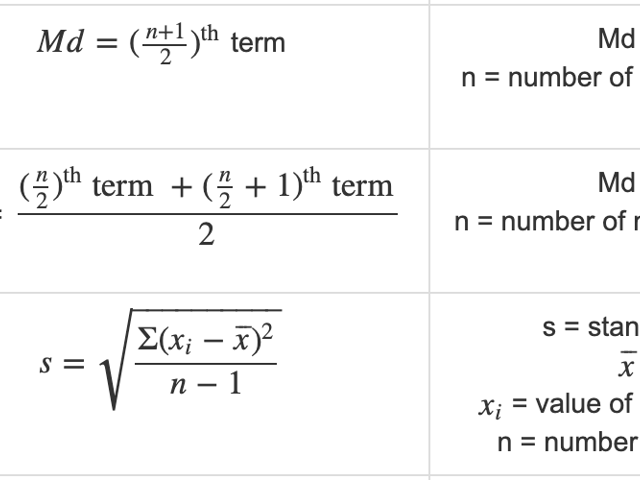# Formulas for the ACT® Science Assessment

The ACT® Science Assessment will test your skills to the limit!

## Which skills?

Every skill you’ve acquired at school! From reading to math, including understanding graphs, evaluating models, and evaluating conflicting viewpoints. You’ll need everything if you want to ace the ACT® Science Assessment.

For this test, you won’t need to memorize anything, because all the necessary information will be given to you. However, knowing the necessary formulas for solving the questions at this test will become handy, so here is a formula chart with useful formulas you can use to practice solving our FREE sample test at Union Test Prep.

Category Formula Symbols Comment
ACT $$\circledR$$
Science
Assessment
$$Du = Su \cdot \frac{Du}{Su} \cdot Su \cdot CF$$ Du = Desired Unit
Su = Starting Unit
CF = Conversion Factor
Multiple steps
may be needed.
ACT $$\circledR$$
Science
Assessment
$$a \cdot b \% = a \cdot \frac{b}{100}$$ a = any real number
b% = any percent
Remember to
simplify.
(if possible)
ACT $$\circledR$$
Science
Assessment
$$\% = \dfrac{ \lvert b-a \rvert}{b} \cdot 100 = \dfrac{c}{b} \cdot 100$$ % = % increase or decrease
a = new value
b = original value
c = amount of change

ACT $$\circledR$$
Science
Assessment
$$rg = lv - sv$$ rg = range
lv = largest value in the data set
sv = smallest value in the data set

ACT $$\circledR$$
Science
Assessment
$$\overline{x} = \dfrac{\Sigma x_i}{n}$$ $$\overline{x}$$ = mean
$$x_i$$ = value of each measurement
n = number of measurements

ACT $$\circledR$$
Science
Assessment
$$Md = (\frac{n+1}{2}) ^{\text{th}}$$ term Md = Median
n = number of measurements (odd)

ACT $$\circledR$$
Science
Assessment
$$Md = \dfrac{(\frac{n}{2})^{\text{th}} \text{ term } + (\frac{n}{2} + 1)^ {\text{th}} \text{ term}}{2}$$ Md = Median
n = number of measurements (even)

ACT $$\circledR$$
Science
Assessment
$$s = \sqrt{\dfrac{ \Sigma (x_i - \overline{x})^2}{n-1}}$$ s = standard deviation
$$\overline{x}$$ = mean
$$x_i$$ = value of each measurement
n = number of measurements

ACT $$\circledR$$
Science
Assessment
$$V= s^2$$ V = Variance
s = standard deviation

ACT $$\circledR$$
Science
Assessment
$$CV = RSD = 100 \cdot \dfrac{s}{\overline{x}}$$ CV = Coefficient of variation
RSD = Relative standard deviation
s = standard deviation
$$\overline{x}$$ = mean